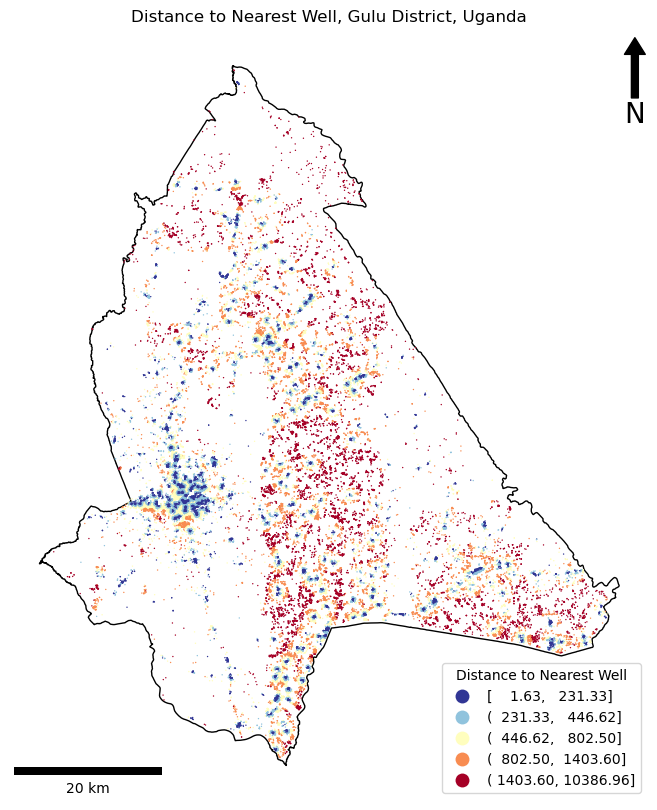# Practical 3 Code Solution``````Initial wells: 3000
Potential wells: 1458
Filtered wells: 975
Minimum distance to water in Gulu District is 2m.
Mean distance to water in Gulu District is 863m.
Maximum distance to water in Gulu District is 10387m.
``````
``````"""
Understanding GIS: Practical 3
@author jonnyhuck

Use spatial index to calculate the mean distance to a water supply in Gulu District

NB: On the rtree 'performance' page, it recommends using a generator function, rather than insert() and a loop,
and also to use objects='raw' in queries. In actual fact, it is faster to use insert and load no object at all
into  the spatial index, then use the .iloc[] function from pandas to retrieve the data. The difference is
c.0.7 seconds vs c.3.1 seconds to complete stage 1 of this practical.

In this exercise, we use an `rtree` implementation of a spatial index rather than the `geodataframe.sindex` one; we implement
Pythagoras' theorem instead of using `geodataframe.geometry.distance()`; and we use a `for` loop and `geodataframe.iterrows()`
instead of `geodataframe.apply()` to create our distance column. These decisions are made  in support of the course's general
aim to follow an  Object Oriented, rather then Functional, programming paradigm; and our related goal of allowing students
to *understand* how these things work - rather than simply being able to use high level functions to achieve the same result,
but without the detailed understanding.

References:
Guttman (1984):	https://dl.acm.org/doi/abs/10.1145/602259.602266
https://epsg.io/21096
http://geopandas.org/reference.html
https://toblerity.org/rtree/examples.html
https://toblerity.org/rtree/tutorial.html#query-the-index
https://matplotlib.org/3.1.1/api/_as_gen/matplotlib.pyplot.legend.html
https://matplotlib.org/3.3.3/tutorials/intermediate/legend_guide.html
https://geopandas.org/en/stable/docs/reference/api/geopandas.GeoDataFrame.estimate_utm_crs.html

New Topics:
Creating Functions
Spatial Indexes with RTree
Iterating through a GeoDataFrame
"""

from math import sqrt
from rtree.index import Index
from matplotlib.pyplot import subplots, savefig
from matplotlib_scalebar.scalebar import ScaleBar

def distance(x1, y1, x2, y2):
"""
* Use Pythagoras' theorem to measure the distance.
* 	https://en.wikipedia.org/wiki/Pythagorean_theorem
*
* This is acceptable in this case because:
*	- the CRS of the data is a local projection
*	- the distances are short
*  	- computational efficiency is important (as we are making many measurements)
"""
return sqrt((x1 - x2)**2 + (y1 - y2)**2)

# read in the shapefiles, and match up the CRS'

''' STAGE 1 - FILTERING THE DATA WITH A SPATIAL QUERY - EXTRACT ONLY WELLS INSIDE THE DISTRICT '''

# initialise an instance of an rtree Index object
idx = Index()

# loop through each row in the wells dataset and load into the spatial index
for id, well in water_points.iterrows():
idx.insert(id, well.geometry.bounds)

# get the one and only polygon from the district dataset (buffer by 10.5km to account for edge cases)
polygon = gulu_district.geometry.iloc.buffer(10500)

# report how many wells there are at this stage
print(f"Initial wells: {len(water_points.index)}")

# get the indexes of wells that intersect bounds of the district, then extract extract the possible matches
possible_matches = water_points.iloc[list(idx.intersection(polygon.bounds))]
print(f"Potential wells: {len(possible_matches.index)}")

# then search the possible matches for precise matches using the slower but more precise method
precise_matches = possible_matches.loc[possible_matches.within(polygon)]
print(f"Filtered wells: {len(precise_matches.index)}")

''' STAGE 2 - NEAREST NEIGHBOUR - find distance to nearest well for everybody'''

# rebuild the spatial index using the new, smaller dataset
idx = Index()
for id, well in precise_matches.iterrows():
idx.insert(id, well.geometry.bounds)

# declare array to store distances
distances = []

# loop through each water source
for id, house in pop_points.iterrows():

# use the spatial index to get the index of the closest well
nearest_well_index = list(idx.nearest(house.geometry.bounds, 1))

# use the spatial index to get the closest well object from the original dataset
nearest_well = water_points.iloc[nearest_well_index]

# store the distance to the nearest well
distances.append(distance(house.geometry.bounds, house.geometry.bounds,
nearest_well.geometry.bounds, nearest_well.geometry.bounds))

# calculate the mean
mean = round(sum(distances) / len(distances))

# store distance to nearest well
pop_points['nearest_well'] = distances

# output the result
print(f"Minimum distance to water in Gulu District is {round(min(distances))}m.")
print(f"Mean distance to water in Gulu District is {mean}m.")
print(f"Maximum distance to water in Gulu District is {round(max(distances))}m.")

# create map axis object
fig, my_ax = subplots(1, 1, figsize=(16, 10))

# remove axes
my_ax.axis('off')

my_ax.set_title("Distance to Nearest Well, Gulu District, Uganda")

gulu_district.plot(
ax = my_ax,
color = 'white',
linewidth = 1,
edgecolor = 'black',
)

# plot the locations, coloured by distance to water
pop_points.plot(
ax = my_ax,
column = 'nearest_well',
linewidth = 0,
markersize = 1,
cmap = 'RdYlBu_r',
scheme = 'quantiles',
legend = 'True',
legend_kwds = {
'loc': 'lower right',
'title': 'Distance to Nearest Well'
}
)